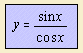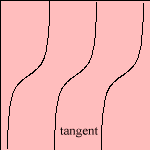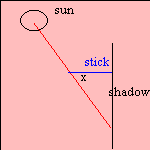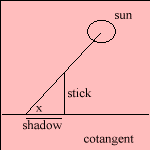# tangent

## trigonometric

last updated: 2004-04-11The tangent can geometrically be defined, in a rectangular triangle, as the proportion of the opposite and the adjacent side. So that tan(x) = sin(x) / cos(x). It has vertical asymptotes for cos(x) = 0.
The old Arabs scholars could derive the height (angle) x of the sun as function of the shadow of a horizontal stick on a vertical plane: they called the shadow, which length is a factor tan(x) of the stick: umbra versa 1).

The tangent rule states a relationship between tan(a+b)/2),   tan((a-b)/2) and the sides a and b.

The cotangent is defined as cot(x) = 1 / tan(x). It is evident that the curve is not more than a tangent that is mirrored in a vertical 2).
The Arab astronomer Al-Battani (900 AD) discovered the cotangent while studying shadows 3). He put a stick in the sand, vertically. He took the stick's shadow as a measure for the height of the sun. The shadow was called the umbra recta or the umbra extensa 4). Itslength is a factor cot(x) of the length of the stick, where x is the angle the sun is to be seen.

The cotangent rule gives cot(a/2) as function of the sides a, b and c.

The inverse functions of the tangent and the cotangent are called the arc tangent arctan(x) and the arc cotangent arccot(x), respectively. In the Netherlands these functions are called the cyclometric functions.
Because the derivative of the arctangent gives the formula of the Cauchy distribution, the arctangent is called the Cauchy cumulative distribution.

The curve resembles the error function.

notes

1) Umbra (Lat.) = shadow, versa (Lat.) = turned.

2) It is easy to see that cot(x) = - tan(x + π/2)

3) Dijksterhuis 1977,  p. 302.

4) Rectus (Lat.) = right, extensa (Lat.) = extended.